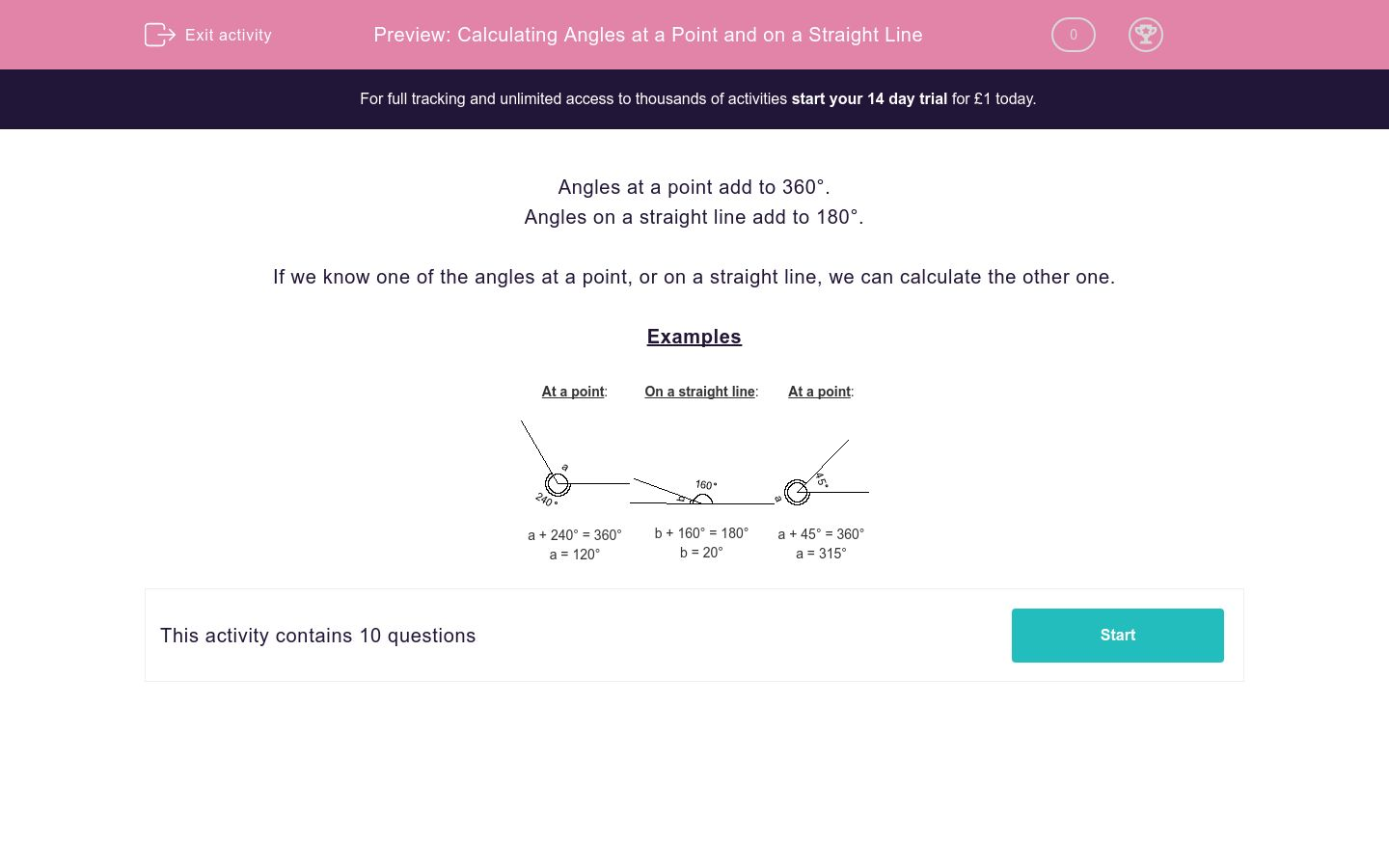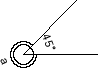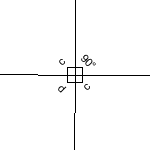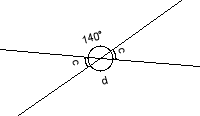# Calculating Angles at a Point and on a Straight Line

In this worksheet, students calculate angles at a point and on a straight line.Key stage:  KS 2

Curriculum topic:   Maths and Numerical Reasoning

Curriculum subtopic:   2D Shapes: Circles, Angles and Bearings

Difficulty level:### QUESTION 1 of 10

Angles at a point add to 360°.

Angles on a straight line add to 180°.

If we know one of the angles at a point, or on a straight line, we can calculate the other one.

Examples

 At a point:a + 240° = 360° a = 120° On a straight line:b + 160° = 180° b = 20° At a point:a + 45° = 360° a = 315°

Calculate the unknown angle.a = 120

a = 120°

a = 60°

Calculate the unknown angle.a = 220°

a = 120°

a = 315°

Calculate the unknown angle.b = 40°

b = 20°

b = 15°

Calculate the unknown angles, c and d.120° 90° 100° c d

Calculate the unknown angle.a = 140°

a = 110°

a = 115°

Calculate the unknown angle.a = 325°

a = 210°

a = 245°

Calculate the unknown angle.a = 85°

a = 90°

a = 45°

Calculate the unknown angle.b = 55°

b = 55

b = 45°

Calculate the unknown angle.b = 55°

b = 180°

b = 25°

Calculate the unknown angles, c and d.140° 60° 40° c d
• Question 1

Calculate the unknown angle.a = 120°
EDDIE SAYS
Angles around a point add up to 360° 360 - 240 = 120
• Question 2

Calculate the unknown angle.a = 315°
EDDIE SAYS
Angles around a point add up to 360° 360 - 45 = 315
• Question 3

Calculate the unknown angle.b = 20°
EDDIE SAYS
Angles on a straight line must add to 180° 180 - 160 = 20
• Question 4

Calculate the unknown angles, c and d.120° 90° 100° c d
EDDIE SAYS
Angles around a point add up to 360° c + 90 = 180
c = 90
d = 90
• Question 5

Calculate the unknown angle.a = 110°
EDDIE SAYS
Angles around a point add up to 360° 360 - 250 = 110
a = 110
• Question 6

Calculate the unknown angle.a = 325°
EDDIE SAYS
Angles around a point add up to 360° 360 - 35 = 325
a = 325
• Question 7

Calculate the unknown angle.a = 85°
EDDIE SAYS
Angles around a point add up to 360° 360 - 275 = 85
a = 85
• Question 8

Calculate the unknown angle.b = 55°
EDDIE SAYS
Angles on a straight line must add to 180° 180 - 125 = 55
b = 55
• Question 9

Calculate the unknown angle.b = 25°
EDDIE SAYS
Angles on a straight line must add to 180° 180 - 155 = 25
b = 25
• Question 10

Calculate the unknown angles, c and d.140° 60° 40° c d
EDDIE SAYS
Angles on a straight line must add to 180° c + 140 = 180
c = 40
d = 180 - 40
d = 140
---- OR ----

Sign up for a £1 trial so you can track and measure your child's progress on this activity.

### What is EdPlace?

We're your National Curriculum aligned online education content provider helping each child succeed in English, maths and science from year 1 to GCSE. With an EdPlace account you’ll be able to track and measure progress, helping each child achieve their best. We build confidence and attainment by personalising each child’s learning at a level that suits them.

Get started